• 邻接矩阵画有向图、无向图邻接矩阵画有向图的逻辑如下： 设邻接矩阵的行(列)数为N，矩阵中非零元素的个数为M，画布宽为W、高为H 1、有向图顶点数为N、有向边数为M，问题转化为：正N边形的所有顶点、以及顶点...
由邻接矩阵画有向图的逻辑如下：
设邻接矩阵的行(列)数为N，矩阵中非零元素的个数为M，画布宽为W、高为H
1、有向图顶点数为N、有向边数为M，问题转化为：画正N边形的所有顶点、以及顶点之间的M条有向线段
2、为方便作图，所有顶点位置相对于画布中心点(W/2, H/2)距离保持一致，该距离可设为一常量R(R>0)
3、两相邻顶点与画布中心点连线之间的夹角互为(2Π/N)
4、设顶点下标为i(0≤i<N)，顶点坐标可表示为(W/2*(1+cos(2Π/N*i+θ)), H/2*(1+sin(2Π/N*i+θ)))(θ为常量，0≤θ<2Π)
5、在画布上：根据顶点坐标画出N个像素点，再根据邻接矩阵非零元素画出这N个点之间的M条有向线段
注：常量R、θ可在有效范围内取任意值，以画出最适合的图形
画无向图的逻辑同上，只是M条有向线段变成(M/2)条无向线段

展开全文• 主要介绍了Python根据已知邻接矩阵绘制无向图操作,涉及Python使用networkx、matplotlib进行数值运算与图形绘制相关操作技巧,需要的朋友可以参考下
• 有向图不对称邻接矩阵无向图对称邻接矩阵 一个简单图实例 import numpy as np # 使用numpy编写的上述有向图的邻接矩阵如下： # 不对称邻接矩阵 A = np.matrix([ [0,1,0,0], [0,0,1,1], [0,1,0,0], [1,0,1,0]...
有向图不对称邻接矩阵变无向图对称邻接矩阵
一个简单图实例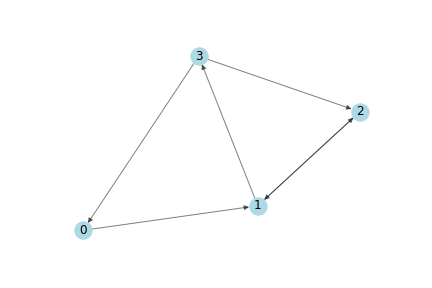import numpy as np

# 使用numpy编写的上述有向图的邻接矩阵如下：
# 不对称邻接矩阵
A = np.matrix([
[0,1,0,0],
[0,0,1,1],
[0,1,0,0],
[1,0,1,0]],
dtype=float
)

A.T

matrix([[0., 0., 0., 1.],
[1., 0., 1., 0.],
[0., 1., 0., 1.],
[0., 1., 0., 0.]])

a = A.T > A
a=a.astype(float)
a

matrix([[0., 0., 0., 1.],
[1., 0., 0., 0.],
[0., 0., 0., 1.],
[0., 1., 0., 0.]])

np.multiply(A.T,a)

matrix([[0., 0., 0., 1.],
[1., 0., 0., 0.],
[0., 0., 0., 1.],
[0., 1., 0., 0.]])

# 变为对称邻接矩阵
A = A + np.multiply(A.T,a) - np.multiply(A,a)
A

matrix([[0., 1., 0., 1.],
[1., 0., 1., 1.],
[0., 1., 0., 1.],
[1., 1., 1., 0.]])



展开全文numpy
• Matlab中根据邻接矩阵做图 function tu_plot(rel,control) ...%第二个输入为控制量，0表示无向图，1表示有向图。默认值为0 r_size=size(rel); if nargin<2 control=0; end if r_size(1)~=r_size(2) di...
Matlab中根据邻接矩阵做图

function tu_plot(rel,control)

%由邻接矩阵画图

%输入为邻接矩阵，必须为方阵；

%第二个输入为控制量，0表示无向图，1表示有向图。默认值为0

r_size=size(rel);

if nargin<2

control=0;

end

if r_size(1)~=r_size(2)

disp('Wrong Input! The input must be a square matrix!');

return;

end

len=r_size(1);

rho=10;%限制图尺寸的大小

r=2/1.05^len;%点的半径

theta=0:(2*pi/len):2*pi*(1-1/len);

[pointx,pointy]=pol2cart(theta',rho);

theta=0:pi/36:2*pi;

[tempx,tempy]=pol2cart(theta',r);

point=[pointx,pointy];

hold on

for i=1:len

temp=[tempx,tempy]+[point(i,1)*ones(length(tempx),1),point(i,2)*ones(length(tempx),1)];

plot(temp(:,1),temp(:,2),'r');

text(point(i,1)-0.3,point(i,2),num2str(i));

%画点

end

for i=1:len

for j=1:len

if rel(i,j)

%连接有关系的点

end

end

end

set(gca,'XLim',[-rho-r,rho+r],'YLim',[-rho-r,rho+r]);

axis off

%%

%连接两点

temp=point2-point1;

if (~temp(1))&&(~temp(2))

return;

%不画子回路；

end

theta=cart2pol(temp(1),temp(2));

[point1_x,point1_y]=pol2cart(theta,r);

point_1=[point1_x,point1_y]+point1;

[point2_x,point2_y]=pol2cart(theta+(2*(theta<pi)-1)*pi,r);

point_2=[point2_x,point2_y]+point2;

if control

arrow(point_1,point_2);

else

plot([point_1(1),point_2(1)],[point_1(2),point_2(2)]);

end

%%

function arrow(start,stop,l)

%start,stop分别为起点和终点

%l为箭头的线长度，默认为主线长的1/10

t=0.1;

ang=15/180*pi;

temp=stop(1)-start(1)+j*(stop(2)-start(2));

L=abs(temp);P=angle(temp);

if nargin<3

l=t*L;

end

p1=P-ang;p2=P+ang;

a=[stop(1)-l*cos(p1) stop(2)-l*sin(p1)];

b=[stop(1)-l*cos(p2) stop(2)-l*sin(p2)];

hold on

plot([start(1) stop(1)],[start(2) stop(2)]);

plot([a(1) stop(1)],[a(2) stop(2)]);

plot([b(1) stop(1)],[b(2) stop(2)]);

end

效果图如下：正在上传…

邻接矩阵为

0     1     0     0     0     0

0     0     0     0     0     1

1     0     0     0     0     1

0     0     1     1     0     1

0     1     0     0     0     1

1     0     0     0     1     0

的有向图：正在上传…


展开全文机器学习
• 这里有一点一定要注意，因为为无向图，因此邻接矩阵一定要是关于对角线对称的，即Aij=Aji（且对角线上元素Aii=0），两点之间相互无向连接，有向图可以不为对称矩阵（有方向） 方法及函数： 1.推荐matlab一个图论很...
这里有一点一定要注意，因为为无向图，因此邻接矩阵一定要是关于对角线对称的，即Aij=Aji（且对角线上元素Aii=0），两点之间相互无向连接，有向图可以不为对称矩阵（有方向）

方法及函数：

1.推荐matlab一个图论很好用的包Graph and Network Algorithms，官网有函数介绍https://www.mathworks.com/help/matlab/graph-and-network-algorithms.html

这里搬一个知乎很不错评论的demo，仅供参考（参考链接文末一起放出）：

%% Matlab 无向图
% 生成数据,A和邻接矩阵的形势相似
A = magic(10);
A = dist(A)；%计算点之间的欧氏距离
index = 1:size(A,1);
names = {};
for ii = index
names{ii} = num2str(index(ii));
end
% 定义图像G
G = graph(A,names)
%% 绘制图像
figure
plot(G,'rs-','LineWidth',2,'MarkerSize',10)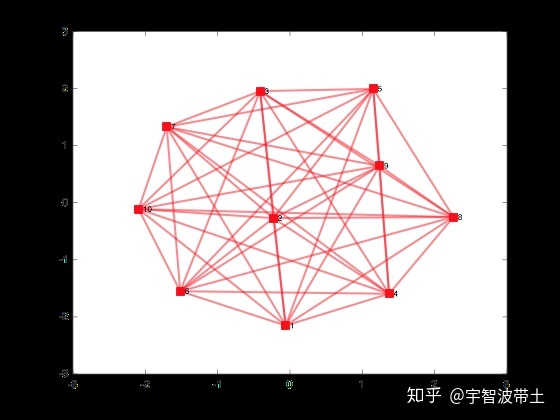还可以改变各个节点之间的绘图方式

%% 绘制图像 定义图像的图层为圆形
figure
plot(G,'ro-','Layout','circle','LineWidth',2,'MarkerSize',10)
<img src="https://pic1.zhimg.com/50/v2-96c490f5c4126fa150e5bc56688bc6db_hd.jpg" data-caption="" data-size="normal" data-rawwidth="560" data-rawheight="420" data-default-watermark-src="https://pic3.zhimg.com/50/v2-e4b7648f26b717dba1f56b58662e9698_hd.jpg" class="origin_image zh-lightbox-thumb" width="560" data-original="https://pic1.zhimg.com/v2-96c490f5c4126fa150e5bc56688bc6db_r.jpg"/>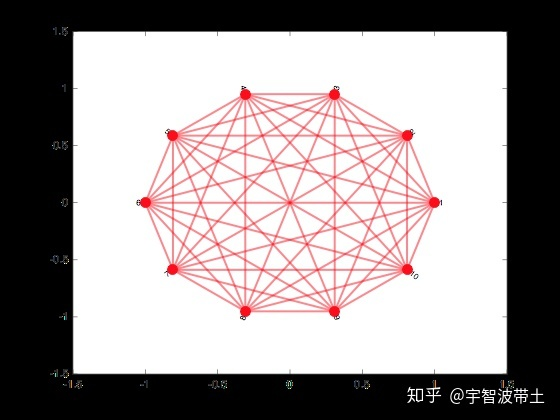如果每个节点是有坐标的，也可以指定节点在空间中的位置

%% 指定每个点的坐标
x = randperm(10)
y = randperm(20,10)
figure
p = plot(G,'ro-','LineWidth',2,'MarkerSize',10)
p.XData = x
P.YData = y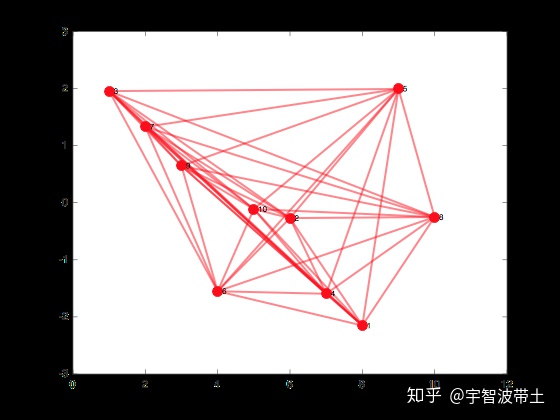2.使用gplot（）

gplot(A, xy):
A表示邻接矩阵，xy表示每个点的位置

如下定义一个函数 netplot，将每个点均匀地放置在单位圆上以画出邻接矩阵：

function netplot(G)
n = size(G, 1);
[x y] = pol2cart((0:n-1)*2*pi/n, 1);
gplot(G, [x' y'], '-o');
end

[X,Y] = POL2CART(TH,R)将极坐标(角度TH和半径R)存储的数据中相应的元素转换到笛卡儿坐标X,Y。数组TH和R必须大小相同(或者二者皆可标量)，TH必须是弧度值。

[X,Y,Z] = POL2CART(TH,R,Z)将圆柱坐标(角度TH、半径R和高度Z)存储的数据中相应的元素转换到笛卡儿坐标X,Y，Z。数组TH、R和Z必须大小相同(或者其中任何一个皆可标量)，TH必须是弧度值。

调用如示：

a = [0 1 1 0; 1 0 1 0; 1 0 0 1; 0 0 1 0];
netplot(a);

输出如图所示：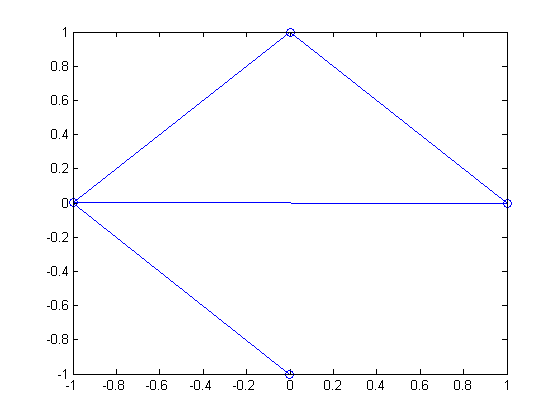参考网址：

https://www.zhihu.com/question/275337007

https://zhidao.baidu.com/question/680827699342938012.html

展开全文机器学习
• 基于邻接矩阵无向图的构建2.深度优先遍历3.广度优先遍历伪代码：构建：1.初始化标志数组2.输入顶点数、边数、顶点信息、边的信息3.初始化arc、vertex深度优先遍历（栈）1.输出一个顶点v并将其标志为已遍历2.递归...深度遍历 广度遍历
• 假设我们要创建一个无向图邻接矩阵 #include<iostream> #define OK 1 #define ERROR 0 #define MaxNum 100 //最大顶点数 typedef char VexType; //顶点数据类型为char型 typedef int VarType; //边的...
• 网上查了很多资料，发现主要是使用邻接表来实现图，并进行遍历的。而采用邻接矩阵的就非常少...不想看的可以直接下载：python 邻接矩阵三种方法实现有向图、无向图，并绘图显示不废话。上代码首先图类 class Graph_Matrpython 二维数组 显示
• 如何用邻接矩阵判断无向图的连通性？ 要Java或者C#的实现思路，最好有代码。
• ## 邻接矩阵无向图

千次阅读 2017-12-29 21:15:58
无向图和有向图在邻接矩阵中的表示方法：  无向图和有向图大同小异，在这里只以无向图为例，代码部分通过简单调整即可对应编译有向图 邻接矩阵数据类型定义 #define MaxVertices 100 //定义最大容量 ...
• 邻接矩阵表示法表示，除了用一个存储邻接矩阵的二维数组外，还需要用一个一维数组来存储顶点信息。 //采用邻接矩阵表示法创建无向网 #include <iostream> using namespace std; #define MaxInt 32767 /...算法 数据结构 c++
• #include&lt;iostream&gt; using namespace std; const int MaxSize=10; class MGraph {public: MGraph(int n,int e){vertexNum=n;arcNum=e; for(int i=0;i&lt;vertexNum;i++){cout&......
• 邻接矩阵实现无权无向图、带权无向图 java 1.图的接口 import java.util.List; public interface Graph { public int getSize(); //返回图中的顶点数 public List getVertices(); //返回图形中的顶点 public V ge...java
• 使用邻接矩阵存储下图所示无向图 **  ** 解题思路  创建一个邻接矩阵 程序实现 #include #include #define MAXVEX 10 /* Status是函数的类型,其值是函数结果状态代码，如OK等 */ typedef int Status;...
• 图的创建及深度广度遍历的代码实现，基于的是邻接矩阵，图这里是无向图，并且两个顶点之间有连接则邻接矩阵非零，如果没有连接则为零 public class Graph { //图的邻接矩阵形式 private int[][] edges; private ...数据结构
• 创建邻接矩阵图的结构体 typedef struct graph { int vexnum,arcnum;//节点个数，弧的个数 int tyust[MAX][MAX];//使用二维数组定义一个矩阵 char vexs[MAX];//存储节点数据 }*Graph; 创建邻接矩阵图 Graph ...
• /*********************邻接矩阵实现无向图******************/ class MatrixUDG { private: char mVexs[MAX];//顶点集合 int mVexBum;//顶点数 int mEdgNum;//边数 int mMatrix[MAX][MAX];//邻接矩阵 char ...c语言
• #include &lt;iostream&gt;#include&lt;stdlib.h&gt;#include&lt;stdio.h&...#define MVNum 100using namespace std;#define STACK_INIT_SIZE 100#define STACKINCREMENT 10#define MAXQSIZE ...广度优先遍历 c++
• /* '邻接矩阵' 实现无向图的创建、深度优先遍历*/ #include <stdio.h> #include <stdlib.h> #define MaxVex 100 //最多顶点个数 #define INFINITY 32768 //表示极大值，即 ∞ #define TRUE ...
• //环境：vs2010 //MGraph.h #ifndef MGraph_H //定义头文件 #define MGraph_H const int MaxSize = 10; //中最多顶点个数 template &lt;class DataType&gt; class MGraph { public: MGraph(...
• #include #define Maxsize 50 #define M 5000//定义无穷数值为5000 typedef struct { char vex[Maxsize];...//矩阵表 int numVertexes,numEdges;//顶点数和边数 }MGraph; void GreateGraph(MGraph *
• //采用邻接矩阵表示法，构造无向图 int i, j, k; cout "输入图的顶点数和边数：" ; cin >> G.vexnum >> G.arcnum; cout "输入各个顶点的名称：" ; for (i = 0 ; i; i++) cin >> G.vexs[i]; ...存储 遍历 class namespace
• 邻接矩阵无向图是指通过邻接矩阵表示的无向图。 上面的图G1包含了"A,B,C,D,E,F,G"共7个顶点，而且包含了"(A,C),(A,D),(A,F),(B,C),(C,D),(E,G),(F,G)"共7条边。由于这是无向图，所以边(A,C)和边(C,A)是同一条边...
• 可考虑用一维数组来存储的结点信息，二维数组来存储节点间的关系，这种存储方式就叫做邻接矩阵的结点结构定义： typedef struct { char vexs[ MVNum ] ;//存储结点信息 int arcs[ MVNum ][ MVNum ] ; //...数据结构 c语言 算法 dfs
• 假设用邻接矩阵储存无向图 设计算法 求出度数最大的顶点编号 急求帮助技术 算法 设计 软件
• 大神：http://blog.csdn.net/esonjohn/article/details/52403185
• import numpy as np from scipy.sparse import coo_matrix adj = coo_matrix((np.ones(5), ([3, 4, 0, 2, 1], [0, 2, 1, 4, 3])), shape=(5, 5), dtype=np.float32) adj = adj + adj.T.multiply(adj.T >...
• 数据结构实验之图论二：图的深度遍历Time Limit: 1000MS Memory Limit: 65536KBSubmit Statistic DiscussProblem Description请定一个无向图，顶点编号从0到n-1，用深度优先搜索(DFS)，遍历并输出。遍历时，先...C语言 算法 数据结构 遍历...